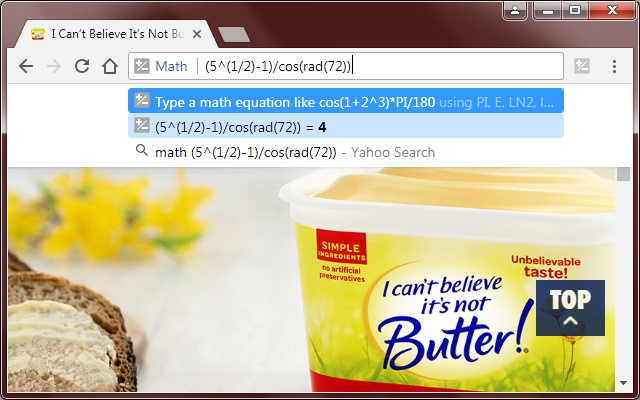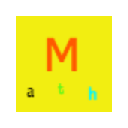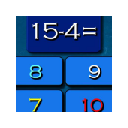# Math

Description from extension meta Solve math equations in the address bar by typing 'math' then something like: (sqrt(5)-1)/cos(rad(72))
Image from storeDescription from store First type "math", then an empty space, then the equation. The answer will appear in the drop down menu. You can type trigonometry too like: math 10 + cos(rad(45)) + log(10) + PI*180 / (2^4 + atan(1)) If you press the "enter" key the result will be saved and is accessible by clicking on the toolbar icon. Only the last 30 calculations will be saved and they will be cleared when the browser is closed. Note that (^) is a "the power of" operator, and not a bitwise-or operator. And that (~) is the "negative" operator, so negative 2 is "~2" and not "-2". Supported constants are: Euler's constant (E), Natural log of 2 (LN2), Natural log of 10 (LN10), Base 2 log of E (LOG2E), Base 10 log of E (LOG10E), Pi (PI), Square root of 0.5 (SQRT1_2), Square root of 2 (SQRT2) Supported (one argument) functions are: abs(x), acos(x), acosh(x), asin(x), asinh(x), atan(x), atanh(x), cube root (cbrt(x)), cos(x), cosh(x), radians to degrees (deg(x)), E to the power of x (exp(x)), log(x), log10(x), log2(x), degrees to radians (rad(x)), sin(x), sinh(x), square root (sqrt(x)), tan(x), tanh(x).

Extpose is a service for Chrome extension publishers.
It helps tracking and optimizing browser extension performance in Chrome Web Store.

The most valuable features are available after creating an account.

If you are not an extension developer and want to install this extension please proceed to Chrome Web Store

## Statistics

Installs
157
Category
Rating
Last update / version
2017-09-04 / 0.1

...
...

## Similar extensions

1Math Hunters: Productivity & Math Helper
79
2Math Practice and Games from kid
524
3Ramanujan - Math Bot
130
4MathHook
101
5Blackboard Grade Grapher
20
6EquatIO - Math made digital
1,716,279
7Pinboard.in: Better Keyboard
53
8Math Evaluation
275
9Subtraction Math Challenge
8
10New Math
24